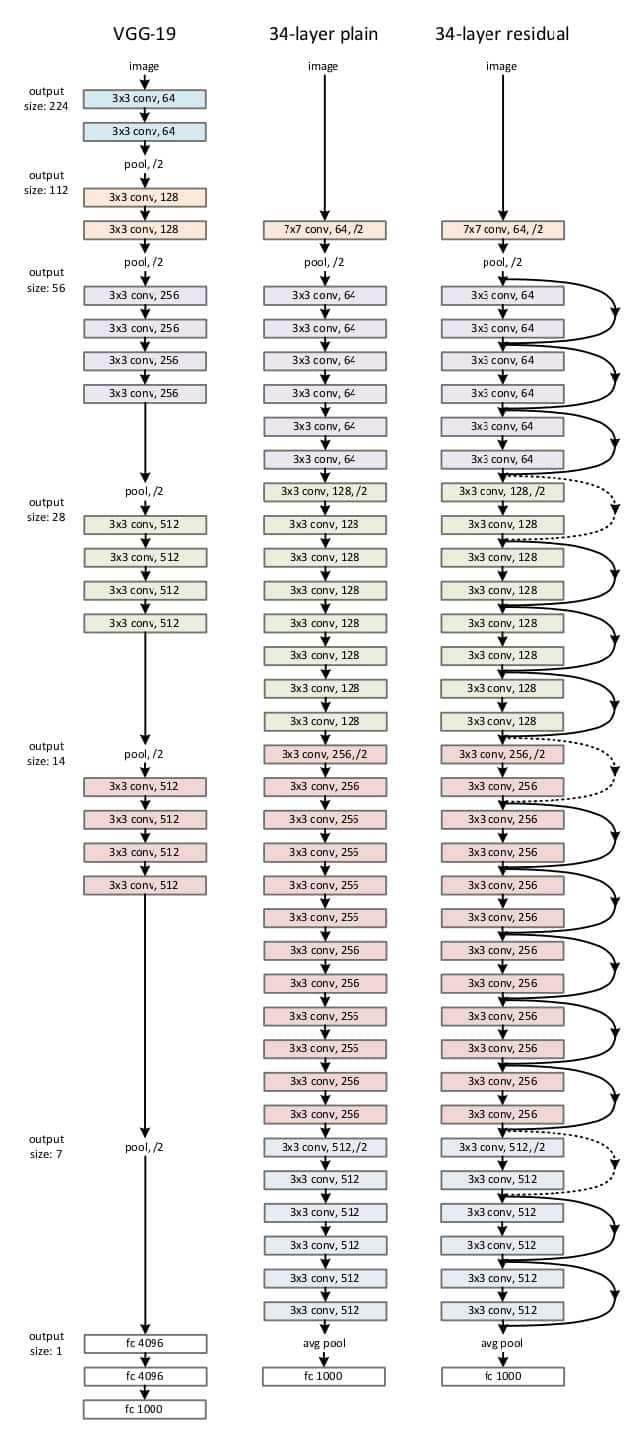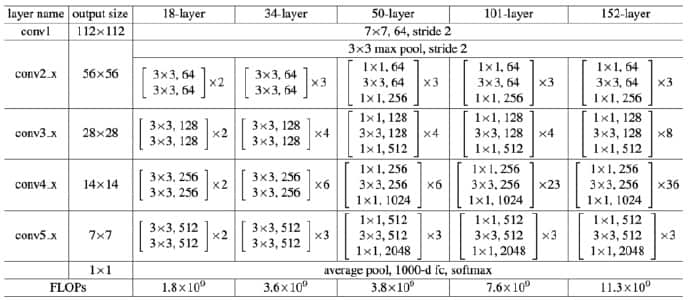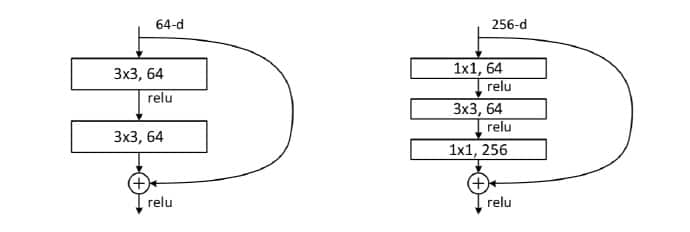ResNet的提出是为了解决梯度消失问题吗？

$$F(x) = f_n(…f_3(f_2(f_1(x)*\theta_1 + b) * \theta_2 +b))$$

### Residual Learning

$$y_l = h(X_l) + F(X_l, W_l), X_{l+1} = f(y_l)$$

$$X_L = X_l + \sum\limits_{i=l}^{L-1}F(X_i, W_i)$$

$$\frac{\partial loss}{\partial {X_l}} = \frac{\partial loss}{\partial {X_L}} \cdot \frac{\partial {X_L}}{\partial {X_l}} = \frac{\partial loss}{\partial {X_L}} \cdot \lgroup 1 + \frac{\partial}{\partial {X_L}} \sum\limits_{i=l}^{L-1} F(X_i, W_i) \rgroup$$

### Network Architectures

ResNet网络结构是参考了VGG19网络，在其基础上进行了修改，并通过短路机制加入了残差单元，如下图所示。主要的变化体现在ResNet直接使用stride=2的卷积做下采样，并且最后使用global average pooling代替了全连接层。ResNet的一个重要的设计原则是：feature map大小降低一半时，feature map的数量增加一倍，这就保持了网络层的复杂度。从上图可以看出，ResNet相比普通网络每两层之间增加了短路机制，这就形成了残差学习。其中虚线表示feature map数量发生了变化。### Summary

ResNet的提出并不是为了解决梯度消失问题，因为当时梯度消失已有BN等方式可以缓解了。
Resnet提出的背景是，更深层的网络不应该比浅层网络差，至少也应该是一样的，因为可以恒等映射。但事实上，对于平原网络结构，更深层的网络结果可能是更糟糕的，论文里有证明了这一点。

1. 这就和上面讨论的，如果只是简单的恒等映射，深层网络至少不应比浅层网络差，可能的问题便是学习的难易程度。